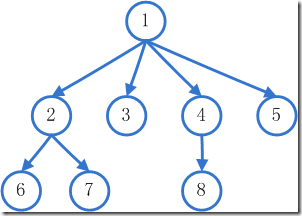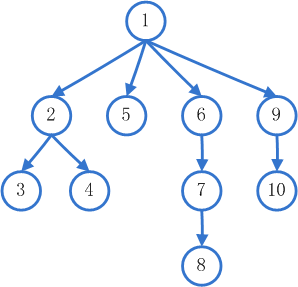# JS算法之A*（A星）寻路算法

BFS的搜索模式是：从图中某节点v出发，在访问了v之后依次访问v的各个未曾访问过的邻接点，然后分别从这些邻接点出发依次访问它们的邻接点，并使得先被访问的节点的邻接点先于后被访问的节点的邻接点被访问，直至图中所有已被访问的节点的邻接点都被访问到。如果此时图中尚有节点未被访问，则需要另选一个未曾被访问过的节点作为新的起始点，重复上述过程，直至图中所有节点都被访问到为止。DFS的搜索模式是：从某个节点v出发，首先访问该节点，然后依次从它的各个未被访问的邻接点出发深度优先搜索遍历图，直至图中所有和v有路径相通的节点都被访问到。 若此时尚有其他节点未被访问到，则另选一个未被访问的节点作起始点，重复上述过程，直至图中所有节点都被访问到为止。 深度优先搜索是一个递归的过程。### A*估价函数

A*算法最重要的就是他的A*估价函数，用来评估哪个节点离目标点最近，用f(n) = g(n) + h(n)来表示。

f(n)是n节点的估价函数；
g(n)是初始点到n节点的实际代价；
h(n)是n节点到目标点的实际代价；

//曼哈顿估价法
private function manhattan(node:Node):Number
{
return Math.abs(node.x - _endNode.x) * _straightCost + Math.abs(node.y + _endNode.y) * _straightCost;
}

//几何估价法
private function euclidian(node:Node):Number
{
var dx:Number=node.x - _endNode.x;
var dy:Number=node.y - _endNode.y;
return Math.sqrt(dx * dx + dy * dy) * _straightCost;
}

//对角线估价法
private function diagonal(node:Node):Number
{
var dx:Number=Math.abs(node.x - _endNode.x);
var dy:Number=Math.abs(node.y - _endNode.y);
var diag:Number=Math.min(dx, dy);
var straight:Number=dx + dy;
return _diagCost * diag + _straightCost * (straight - 2 * diag);
}### Open和Closed列表

open列表：用来记录当前节点所有可能访问的节点；

closed列表：用来记录估价后被否决掉的节点，以后就不用再对这些节点估价了；

### 工作原理

1. 把与当前节点相邻的节点加入到open列表（若非起始结点，则不包括被上一个节点加入到closed列表的节点）；
2. 算出open列表里所有节点的估价函数f(n)值（总实际代价），得出最小的节点并移动到该节点，还原（清空）open列表；
3. 把第2步否决掉的节点记录到closed列表中；

### 参考文章

[Unity] A-Star(A星)寻路算法

posted @ 2017-07-22 23:51  CHENJIAJIE  阅读(4999)  评论(0编辑  收藏